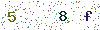﻿ Basic Knoledge of Power Factor - LISUNLISUN

# Basic Knoledge of Power Factor

Power factor shows the capacity of the active power output of lamps and lanterns. Power is the rate of energy passing, and it is the multiply of dc voltage V and current . It is more complicated in communication system: part of the alternating current loop does not transmit power in the load, it is called the reactive current or harmonic current, it makes the apparent power the multiply of voltage and current greater than the actual power. The unequal of apparent power and real power raising power factor which is equal to the ratio of actual power and apparent power. So, in the communication system the actual power is equal to the apparent power multiplied by the power factor.
I.e., the power factor = actual power/apparent power.
Only power factor of linear load , such as electric heater and the bulb, is 1. For many equipments, the difference between actual power and apparent power is quite small, and can be neglected, but for capacitive equipment such as lamps and lanterns, the difference is very big, and also very important. A recent study of the American journal of PC Magazine says that the typical power factor of lamps and lanterns is 0.65 which means the apparent power (VA) is almost 50% larger than the actual power (Watts).